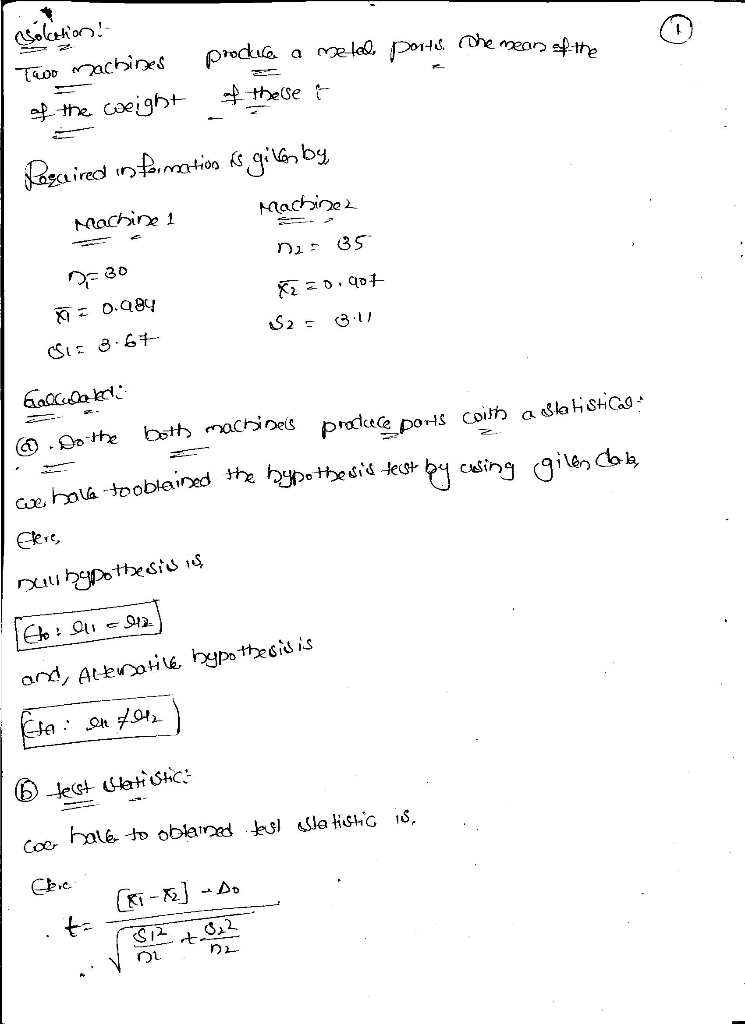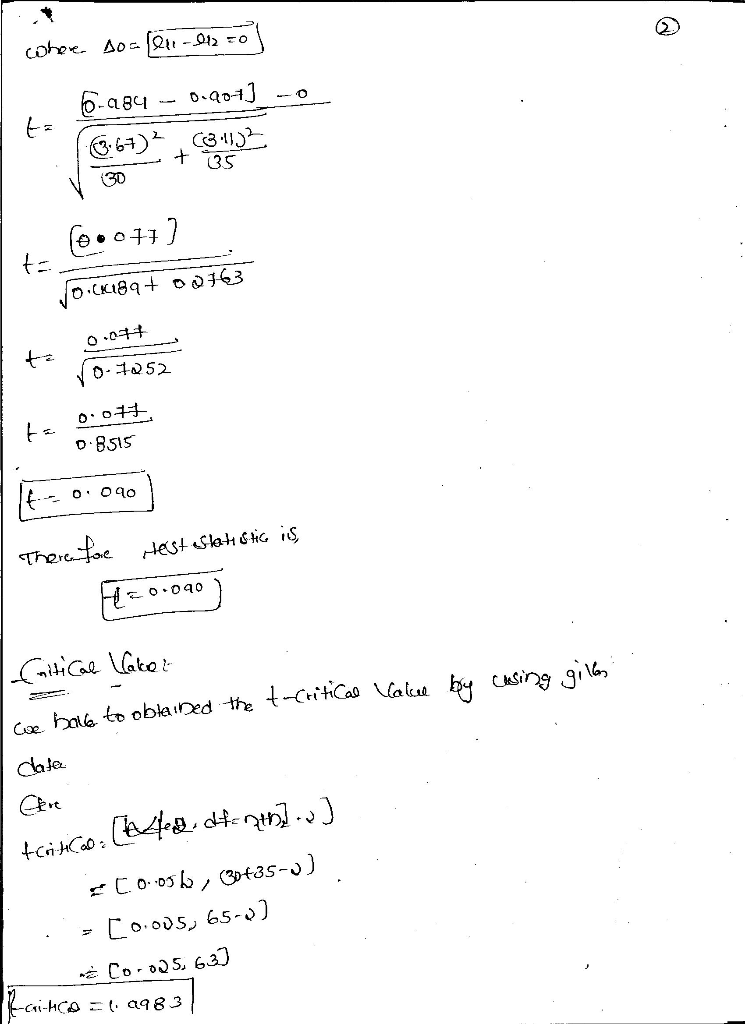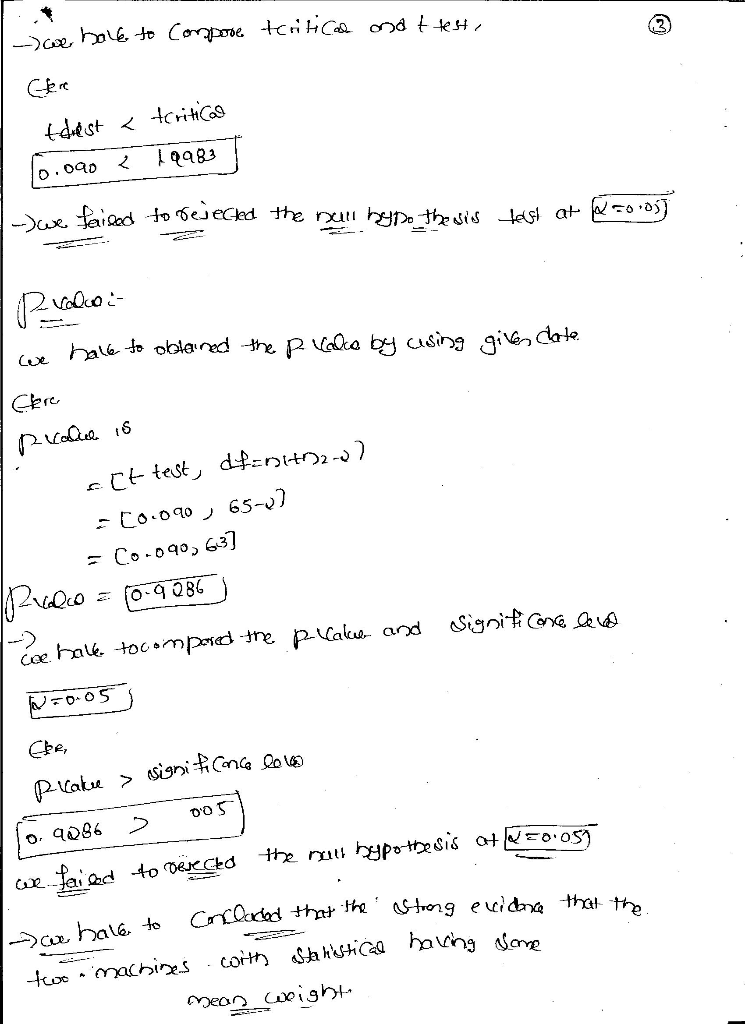Homework Help Question & Answers

# Two machines produce metal parts. The mean of the weight of these parts is of interest. The following data have been collected Machine 1 N 30 x(dash) = 0.984 σ 3.67 Machine 2 n 35 x (dash) 0.907 σ 3.1...

Two machines produce metal parts. The mean of the weight of these parts is of interest. The following data have been collected

Machine 1

N 30

x(dash) = 0.984

σ 3.67

Machine 2

n 35

x (dash) 0.907

σ 3.11

a. Do both machines produce parts with a statistical same mean weight? State your null and alternative hypothesis, and use a 1% level of significance.

b. If we want the confidence interval to have a half width of 0.05, how many pieces should be collected?Due to insufficieint time , i will not answer question (b) .

please send the separate question , i will answer it .

Thank you

##### Add Answer of: Two machines produce metal parts. The mean of the weight of these parts is of interest. The following data have been collected Machine 1 N 30 x(dash) = 0.984 σ 3.67 Machine 2 n 35 x (dash) 0.907 σ 3.1...
More Homework Help Questions Additional questions in this topic.

• #### The Wi total utility costs for the past 6 months ildcat Company has provided the following data on machine the following data on machine-hours wo Month Machine-Hours Total Urility Costs \$ 2,522,4...

Need Online Homework Help?

Get FREE EXPERT Answers
WITHIN MINUTES
Related Questions